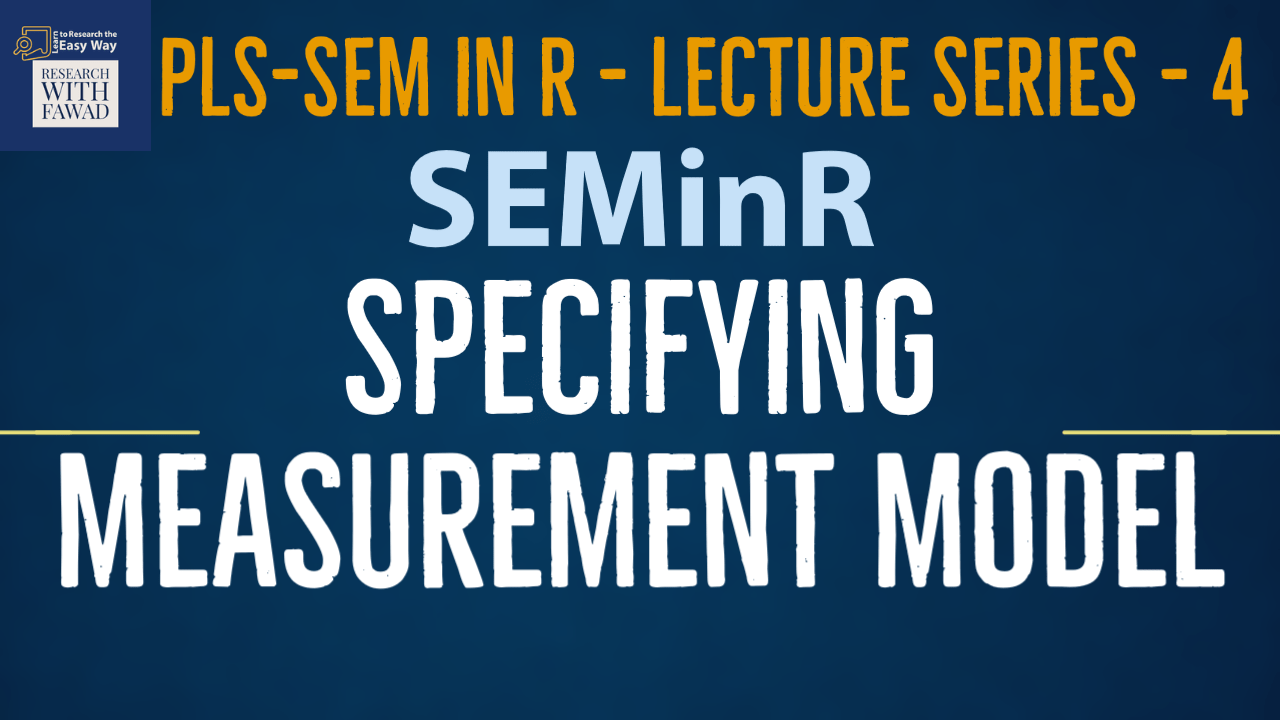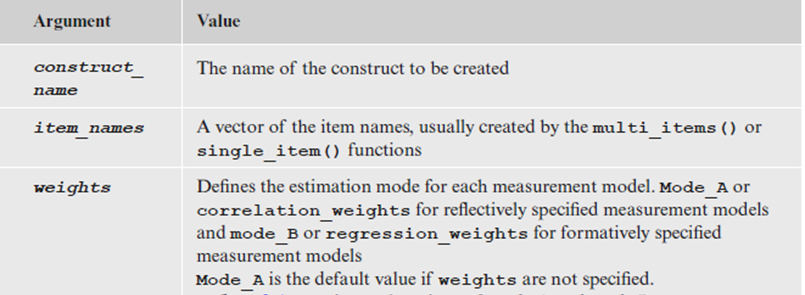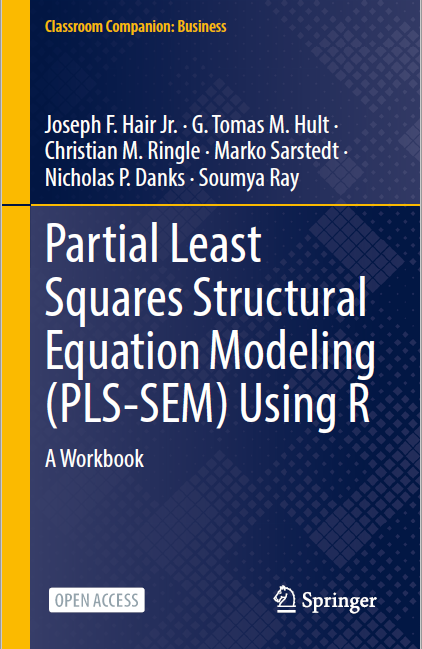# Specifying Measurement Model in SEMinR### Specifying Measurement Model in SEMinR

This lecture on SEMinR Package will introduce How to Specify the Measurement Model in the SEMinR package.

## SEMinR

There are four steps to specify and estimate a structural equation model using SEMinR:

• Specifying the measurement models
• Specifying the structural model
• Estimating, bootstrapping, and summarizing the model

## Step 2: Specify the Measurement Model

• Path models are made up of two elements:
• The measurement models (also called outer models in PLS-SEM), which describe the relationships between the latent variables and their measures (i.e., their indicators), and
• The structural model (also called the inner model in PLS-SEM), which describes the relationships between the latent variables. We begin with describing how to specify the measurement models.
• Measurement model is assessed to establish the quality criteria (Reliability and Validity).
• Hypothesis tests involving the structural relationships among constructs will only be as reliable or valid as the construct measures.
• SEMinR uses the constructs() function to specify the list of all construct measurement models. Within this list, various constructs can be defined using:
• composite() specifies the measurement of individual constructs.
• interaction_term() specifies interaction terms.
• higher_composite() specifies hierarchical component models (higher-order constructs; Sarstedt et al., 2019).
• The constructs() function compiles the list of constructs and their respective measurement model definitions.
• We must supply it with any number of individual composite(), interaction_term(), or higher_composite() constructs using their respective functions.
• The composite() function describes the measurement model of a single construct and takes the arguments shown in Table.• SEMinR strives to make specification of measurement items shorter and cleaner using multi_items(), which creates a vector of multiple measurement items with similar names or single_item() that describes a single measurement item.
• A vector is a sequence of data elements of the same basic type. Members in a vector are officially called components. Vectors in R are the same as the arrays in C language which are used to hold multiple data values of the same type.
• For example, we can use composite() for PLS path models to describe the reflectively measured Constructs

composite(“Put in Construct Name in Quotes”, multi_items(“Construct Code”, Starting Number:Ending Number), weights = mode_A);

Collaborative Culture construct with its indicator variables CC1, CC2, CC3, CC4, CC5, CC6:

• Explanations of mode A and mode B are discussed later. When no measurement weighting scheme is specified, the argument default is set to mode_A.
``composite(“Collaborative Culture”, multi_items(“CC”, 1:6), weights = mode_A);``
• Similarly, if you have a single item construct, you can use composite() to define the single-item measurement model as

composite(“CUSA”, single_item(“cusa”))

• Using composite define your constructs in the mode, next, combine the measurement models within the constructs() function, we can define the measurement model for the simple model like using constructs and composite (see next slide).Note: If an error occurs, make sure you used the library(seminr) command in R to load the SEMinR package before executing the program code.

The program code facilitates the specification of standard measurement models. However, the constructs() function also allows specifying more complex models, such as interaction terms (Memon et al., 2019) and higher-order constructs (Sarstedt et al., 2019). We will discuss the interaction_term() function for specifying interactions in more detail later.

Step 2 in Creating a Model – Identify the variables in your study and Put them as Measurement Model.

``````#Step 2: Create measurement model
simple_mm <- constructs(
composite("Vision", multi_items("VIS", 1:4)),
composite("Development", multi_items("DEV", 1:7)),
composite("Rewards", multi_items("RW",1:4)),
composite("Collaborative Culture", multi_items("CC", 1:6)))``````

Here simple_mm is an object which stores the constructs in the study.

<- Can be considered as an equal sign that assigns the constructs to the object.

constructs function holds the variables from the study, defined as composite (as discussed in the last slide)

## Review of the Steps

Following is a brief review of the steps that have been discussed in SEMinR tutorials.

• Load the Library – library ()
• Review the Data – head()
• Specify the Measurement Model – constructs()

## Reference

Hair Jr, J. F., Hult, G. T. M., Ringle, C. M., Sarstedt, M., Danks, N. P., & Ray, S. (2021). Partial Least Squares Structural Equation Modeling (PLS-SEM) Using R: A Workbook.The tutorials on SEMinR are based on the mentioned book. The book is open source and available for download under this link.